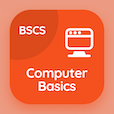Colleges Online Courses

Applied Physics Quizzes

Applied Physics Quiz PDF - Complete

# Modern Physics Multiple Choice Questions p. 107

Study Modern Physics multiple choice questions and answers, modern physics quiz answers PDF 107 to study Applied Physics course online. Modern Physics MCQ trivia questions, modern physics Multiple Choice Questions (MCQ) for online college degrees. "Modern Physics MCQ" PDF eBook: uniformly accelerated motion, physical quantities in physics, electric and gravitational forces, velocity time graph, modern physics test prep for GRE subject tests.

"Compton shift is max for scattering angle of" MCQ PDF: 90, 0, 180, and 45 for best online colleges for teaching degree. Learn modern physics questions and answers to improve problem solving skills for GRE subject test tutoring.

## Modern Physics Questions and Answers MCQs

MCQ: Compton shift is max for scattering angle of

0
90
180
45

MCQ: Area under the velocity-time graph is equal to the

1/2(V × T)
(V × t)
1/2(V + t)
(V + t)

MCQ: Electric force between two charges is similar to the gravitational force between

two point charges
two point masses
two bodies
two distances

MCQ: One of the physical quantities which is not a base quantity is

length
mass
force
time

MCQ: Velocity of the vertically thrown ball with time will be

upwards positive
downwards negative
upwards negative
downwards positive

### More Quizzes from Applied Physics Course